Reconstruction of the 2015 Ox Drove formation 1.Draw a circle. Draw the horizontal and vertical centerlines. 2.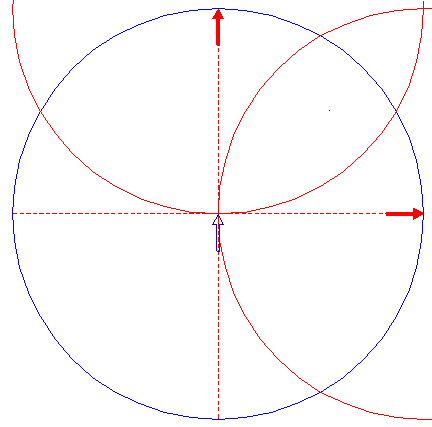Copy circle 1 two times, to its upper and righthand intersections with the horizontal and vertical centerlines. 3.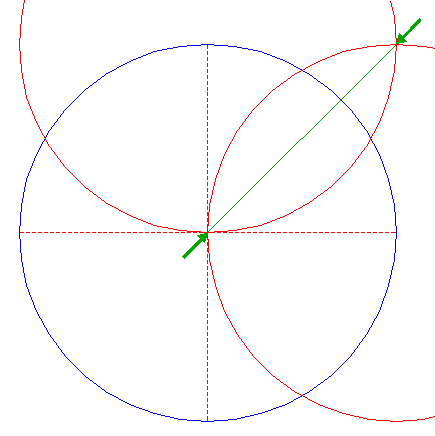Draw the connecting line between the mutual intersections of circles 2. 4.Construct the inscribed dodecagon (regular 12-sided polygon) of circle 1, with one angular point on line 3. Number the angular points clockwise 1 - 12, starting at the upper righthand one. 5.Draw a line between the upper intersection of circle 1 and the vertical centerline and angular point 4 nr. 6. 6.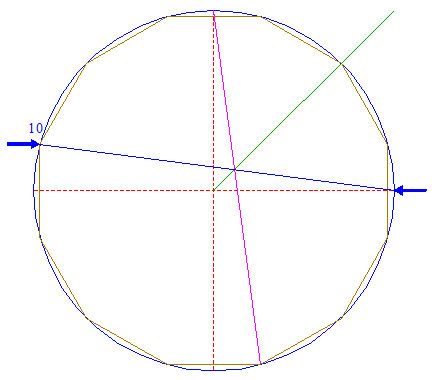Draw a line between the righthand intersection of circle 1 and the horizontal centerline and angular point 4 nr. 10. 7.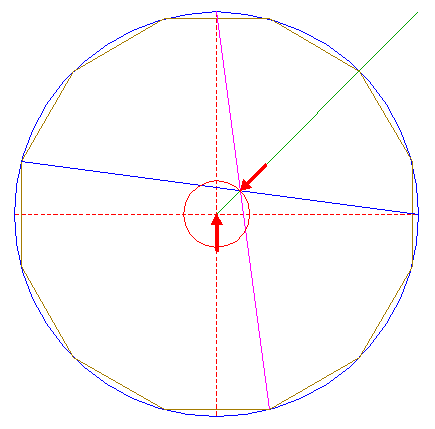Construct a circle concentric to circle 1, passing through the intersection of lines 5 and 6. 8.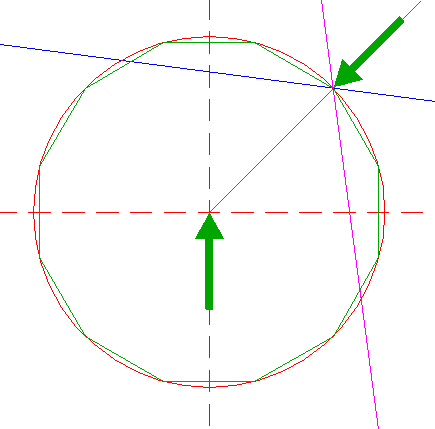Construct the inscribed dodecagon of circle 7, with one angular point on line 3. 9.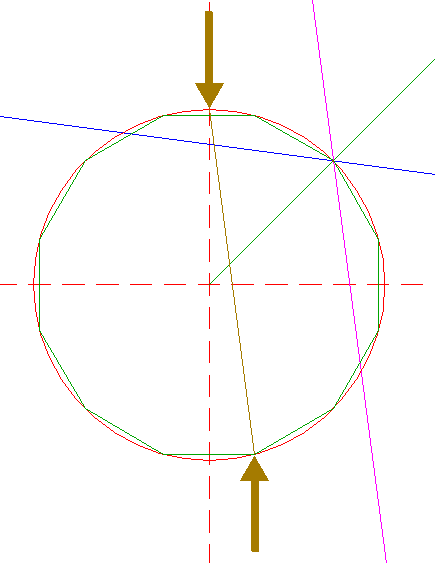Draw a line similar to line 5, with respect to circle 7 and dodecagon 8. 10.Draw a line similar to line 6, with respect to circle 7 and dodecagon 8. 11.Construct a circle concentric to circle 1, passing through the intersection of lines 9 and 10. 12.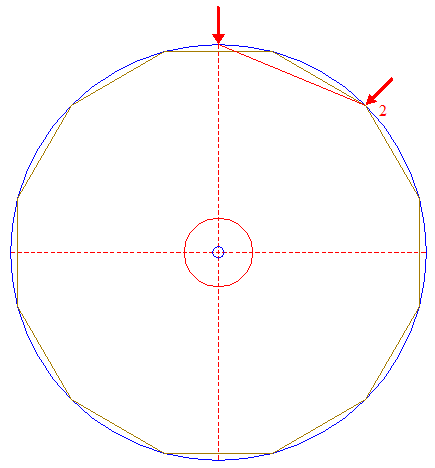Draw a line between the upper intersection of circle 1 and the vertical centerline and angular point 4 nr. 2. 13.Construct a circle concentric to circle 1, tangent to line 12. 14.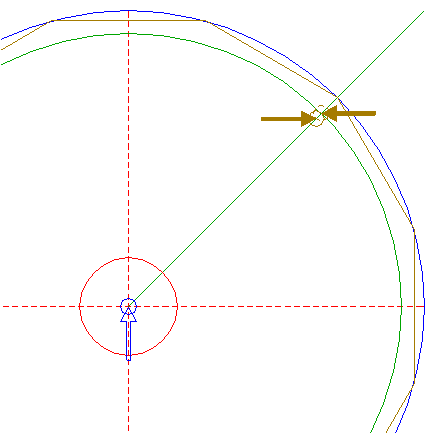Copy circle 11 to the intersection of circle 13 and line 3, and move this circle (copy and delete original) to its own lower lefthand intersection with line 3. 15.Construct a circle concentric to circle 1, tangent to circle 14 at the lower lefthand side. 16.Construct a circle centered at intersection of circle 15 and line 3, tangent to circle 13 at the upper righthand side. 17.Construct a circle concentric to circle 1, tangent to circle 16 at the lower lefthand side. 18.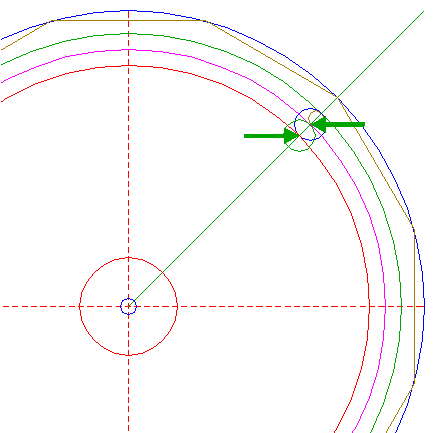Construct a circle centered at the intersection of circle 17 and line 3, tangent to circle 15 at the upper righthand side. 19.Construct a circle concentric to circle 1, tangent to circle 18 at the lower lefthand side. 20.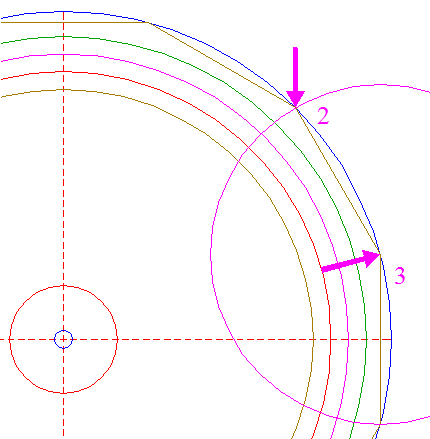Construct a circle centered at angular point 4 nr 3, passing through angular point 4 nr. 2. 21.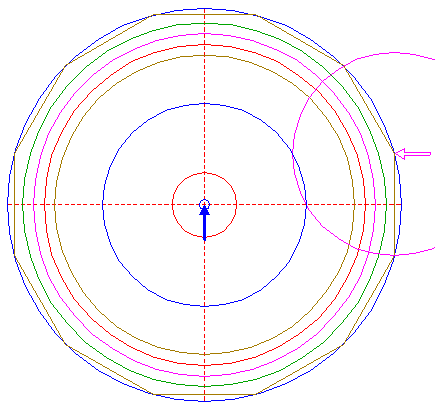Copy circle 20 to the center of circle 1. 22.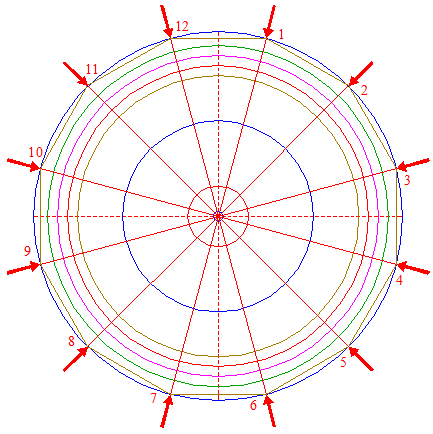Draw the twelve rays of dodecagon 4, from the center of circle 1 to the angular points, and number the rays according to the angular points. 23.Construct a circle centered at the righthand intersection of circle 19 and the horizontal centerline, passing through the intersection of circle 17 and ray 22 nr. 3. 24.Copy circle 23 to the intersection of circle 21 and ray 22 nr. 3. 25.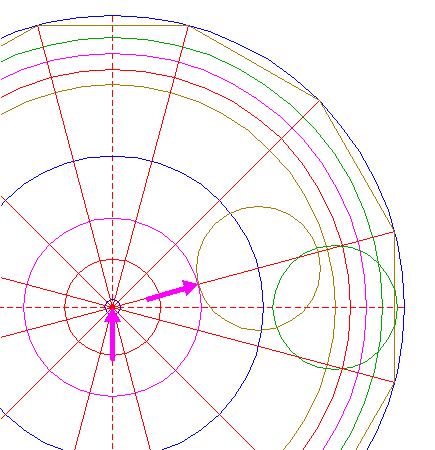Construct a circle concentric to circle 1, tangent to circle 24 at the lefthand side. 26.Copy circle 23 to the intersection of circle 20 and ray 22 nr. 3. 27.Construct a circle concentric to circle 1, tangent to circle 26 at the lefthand side. 28.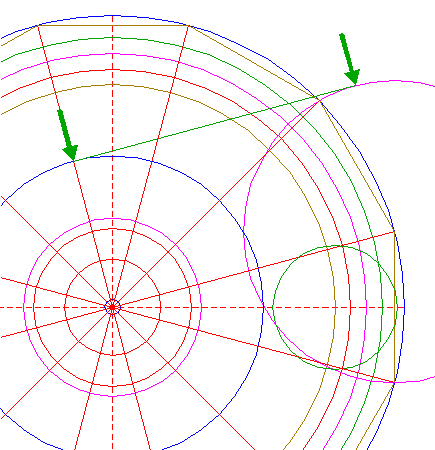Construct a line tangent to circles 20 and 21, both at the upper sides. 29.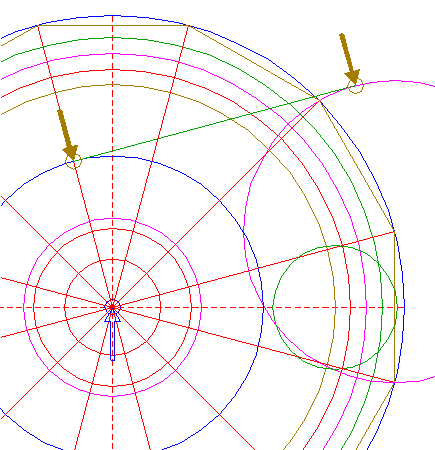Copy circle 11 two times, to the intersections (tangent points) of line 28 and circles 20 and 21. 30.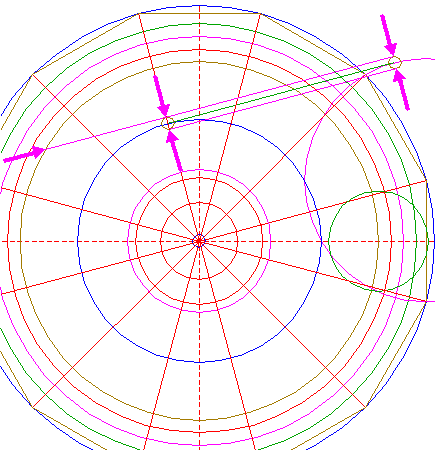Construct two parallel lines, tangent to circles 29 at both sides, and extend the upper one to the left upto circle 19. 31.Construct two parallel lines, tangent to circle 11 and lefthand circle 29 at both sides, and extend the lefthand one upto the upper line 30. 32.Copy circle 23 to the center of lefthand circle 29. 33.Copy circle 11 two times, to the upper intersection of circle 15 and the vertical centerline, and to the intersection of circle 32 and line 28. 34.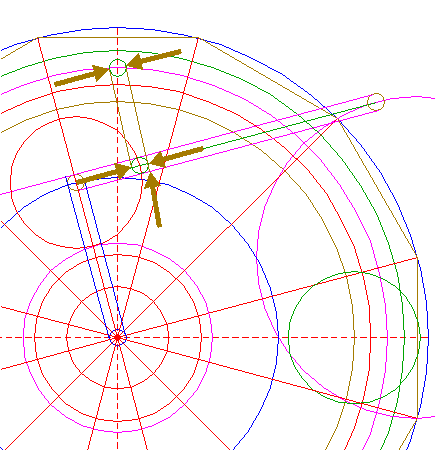Construct two parallel lines, tangent to circles 33 at both sides, and extend the righthand one downto the lower line 30. 35.Remove all redundant parts from lines 30, 31 and 34, and create the pattern as shown. 36.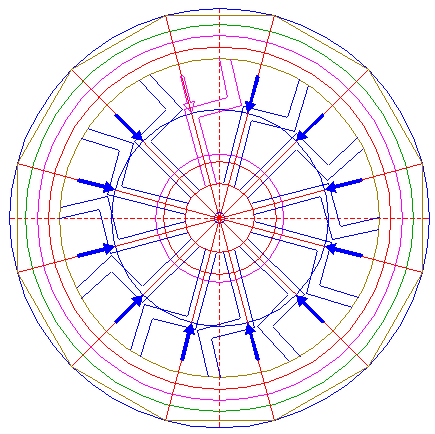Copy pattern 35 eleven times, by repeating all necessary steps with respect to the other angular points 4 (nrs. 1 - 11). 37.Construct a "two-points" circle (defined by the two end-points of a centerline) between the intersections of ray 22 nr. 2 and circles 17 and 19. 38.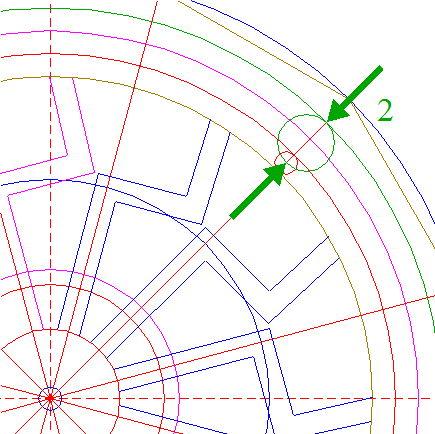Construct a "two-points" circle between the center of circle 37 and the intersection of ray 22 nr. 2 and circle 13. 39.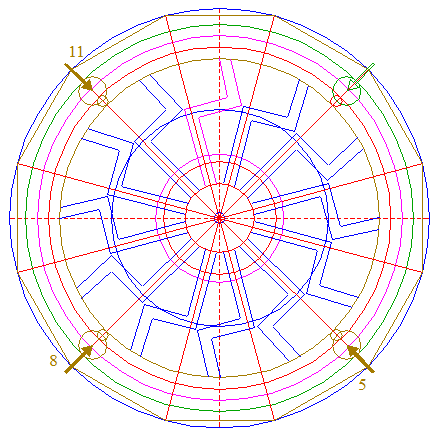Repeat steps 37 and 38 with respect to rays 22 nrs. 5, 8, and 11. 40.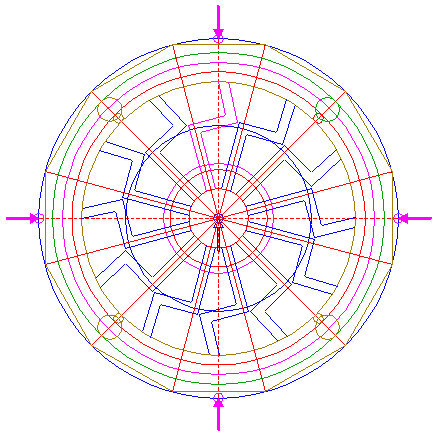Copy circle 11 four times, to the intersections of circle 1 and the horizontal and vertical centerlines. 41.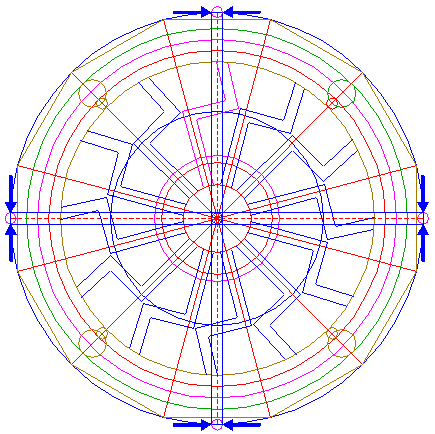Construct two pairs of parallel lines, parallel to the horizontal and vertical centerlines, pairwise tangent to circles 40 at both sides. 42.Circles 1, 7, 13, 15, 17, 19, 25, 27, 38, and 39, lines 22, and 41, and patterns 35 and 36, are used for the final reconstruction. 43.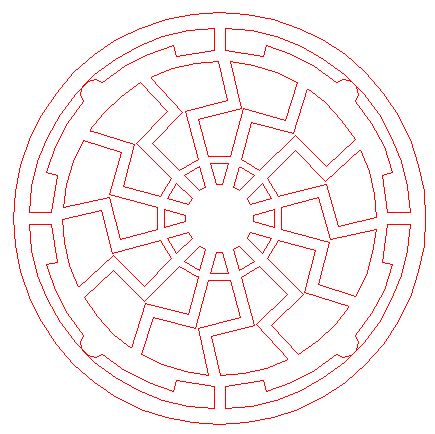Remove all parts not visible within the formation itself. 44.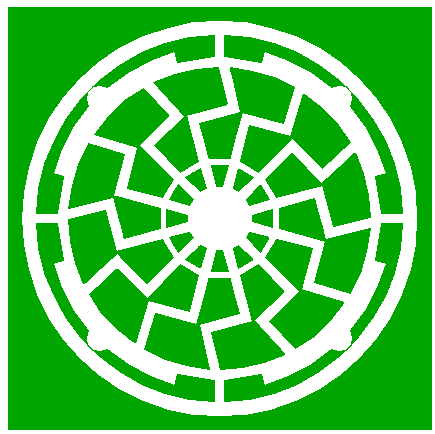Colour all areas corresponding to standing... 45.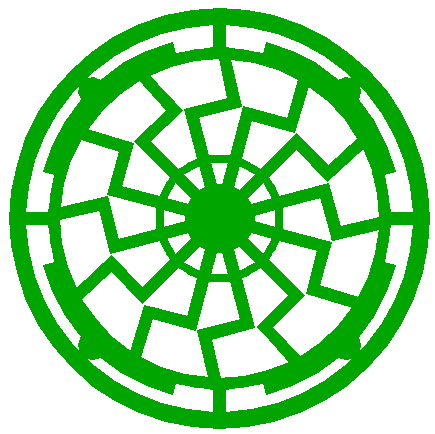...or to flattened crop, and finish the reconstruction of the 2015 Ox Drove formation. 46.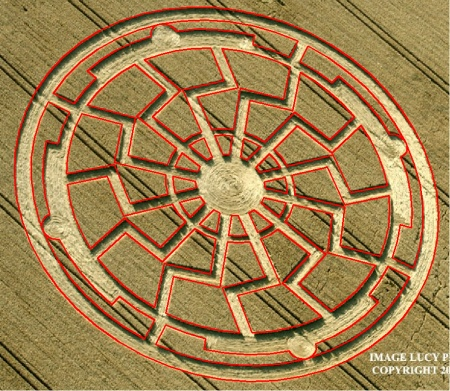The final result, matched with the aerial image.